8 Basic Graphics Objects
In the Wolfram Language, Circle[] represents a circle. To display the circle as graphics, use the function Graphics. Later, well see how to specify the position and size of a circle. But for now, were just going to deal with a basic circle, which doesnt need any additional input.
Make graphics of a circle:
 In:=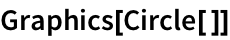Out=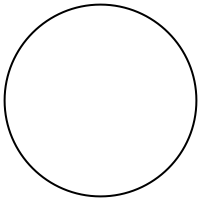Disk represents a filled-in disk:
 In:=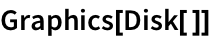Out=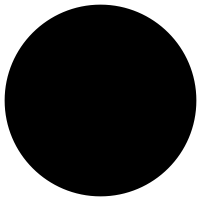RegularPolygon gives a regular polygon with however many sides you specify.
Heres a pentagon (5-sided regular polygon):
 In:=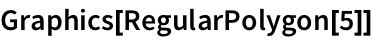Out=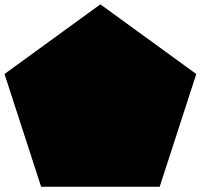In:=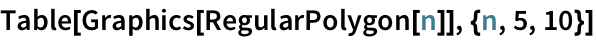Out=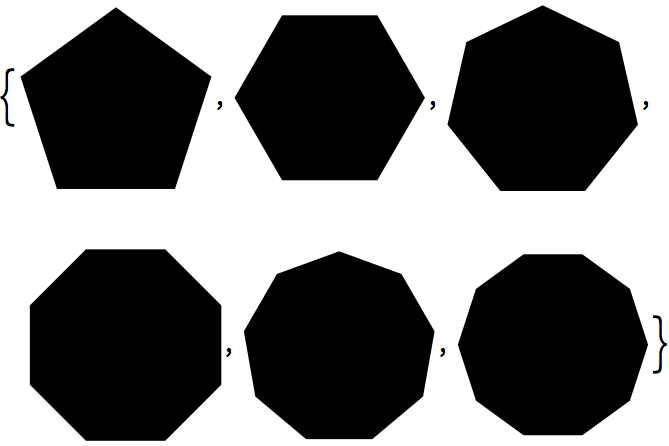Style works inside Graphics, so you can use it to give colors.
Heres an orange pentagon:
 In:=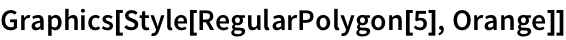Out=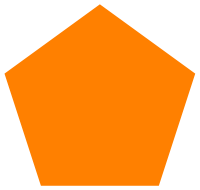The Wolfram Language works in 3D as well as 2D, with constructs such as Sphere, Cylinder and Cone. When you have 3D graphics, you can rotate them around interactively to see different angles.
Display a sphere in 3D:
 In:=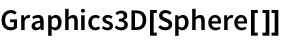Out=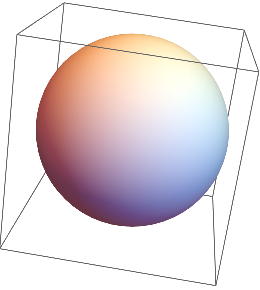A list of a cone and a cylinder:
 In:=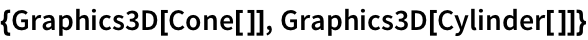Out=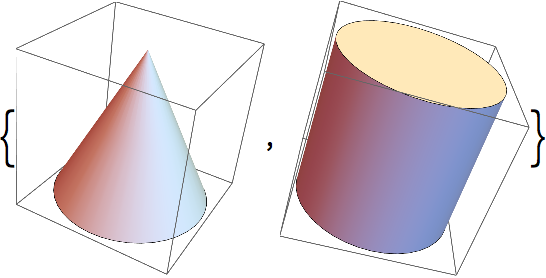A yellow sphere:
 In:=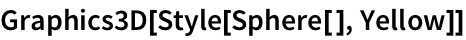Out=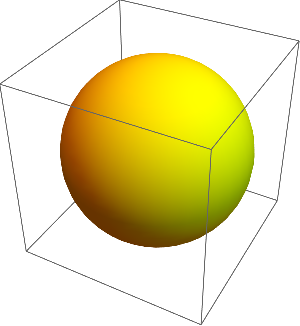Circle[ ] specify a circle Disk[ ] specify a filled‐in disk RegularPolygon[n] specify a regular polygon with n sides Graphics[object] display an object as graphics Sphere[ ], Cylinder[ ], Cone[ ], ... specify 3D geometric shapes Graphics3D[object] display an object as 3D graphics
8.1Use RegularPolygon to draw a triangle. »
Expected output:
 Out[]=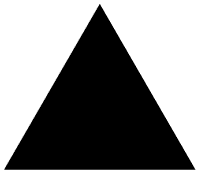Answer & check your solution
8.2Make graphics of a red circle. »
Expected output:
 Out[]=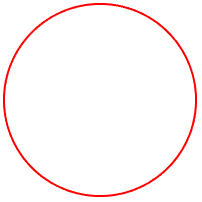Answer & check your solution
8.3Make a red octagon. »
Expected output:
 Out[]=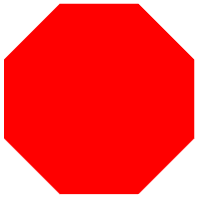Answer & check your solution
8.4Make a list whose elements are disks with hues varying from 0 to 1 in steps of 0.1. »
Expected output:
 Out[]=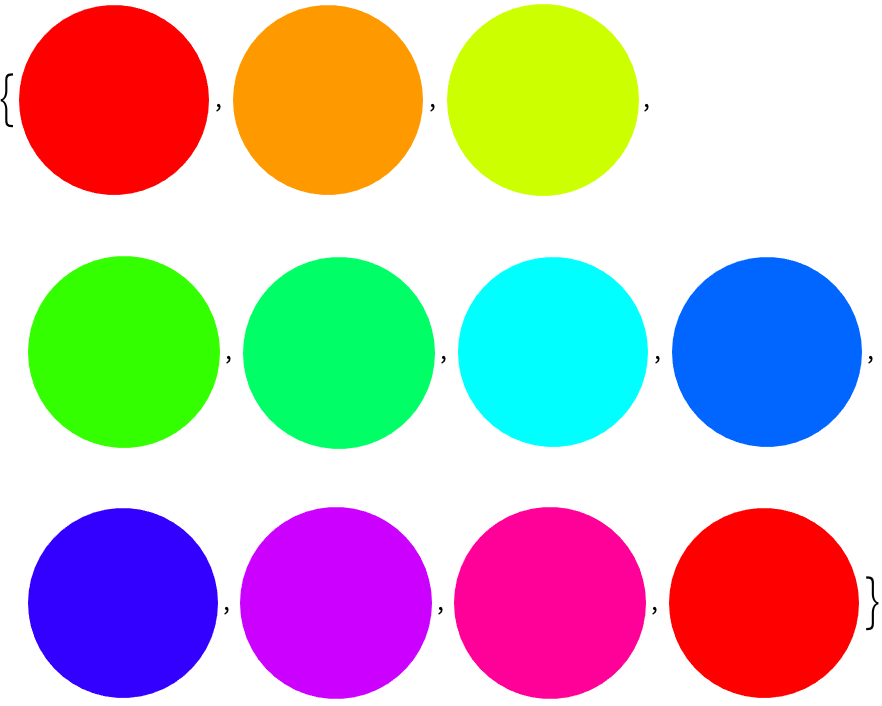Answer & check your solution
8.5Make a column of a red and a green triangle. »
Expected output:
 Out[]=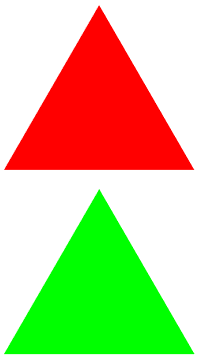Answer & check your solution
8.6Make a list giving the regular polygons with 5 through 10 sides, with each polygon being colored pink. »
Expected output:
 Out[]=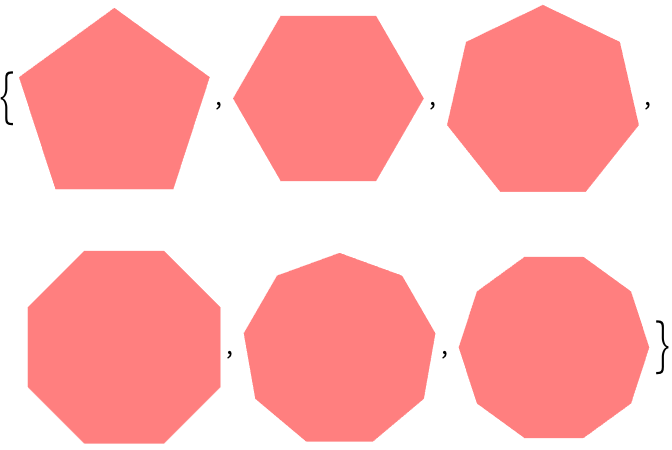Answer & check your solution
8.7Make a graphic of a purple cylinder. »
Expected output:
 Out[]=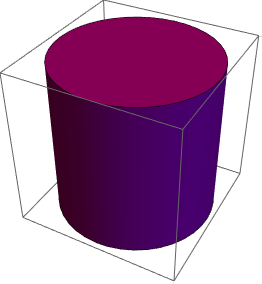Answer & check your solution
8.8Make a list of polygons with 8, 7, 6, ... , 3 sides, and colored with RandomColor, then show them all overlaid with the triangle on top (hint: apply Graphics to the list). »
Sample expected output:
 Out[]=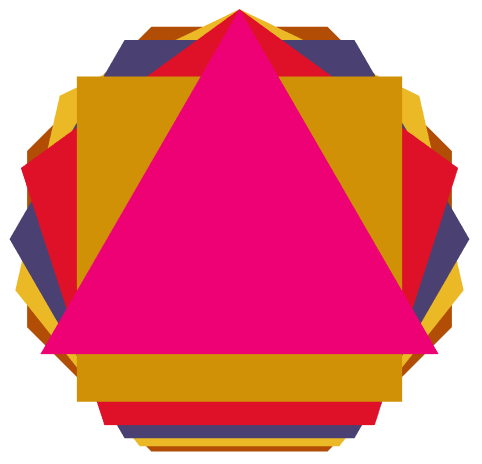Answer & check your solution
+8.1Make a list of 10 regular pentagons with random colors.  »
Sample expected output:
 Out[]=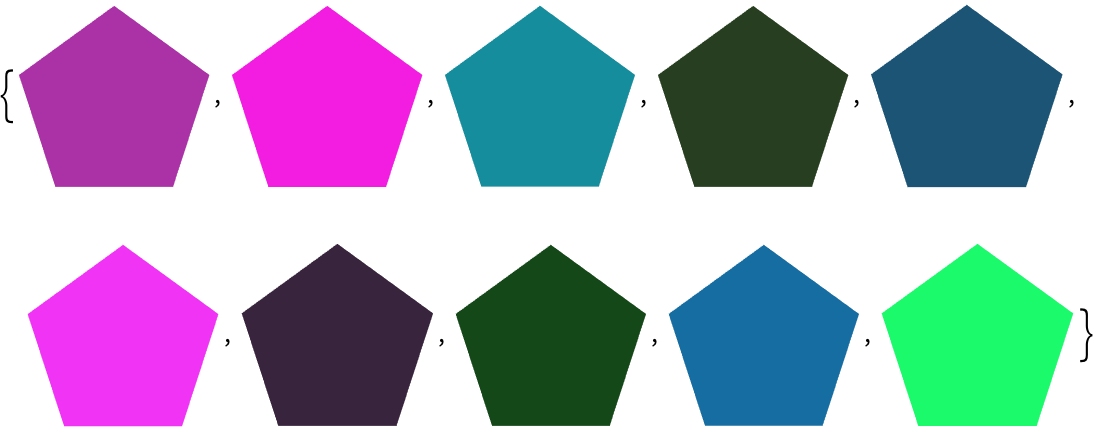Answer & check your solution
+8.2Make a list of a 20-sided regular polygon and a disk. »
Expected output:
 Out[]=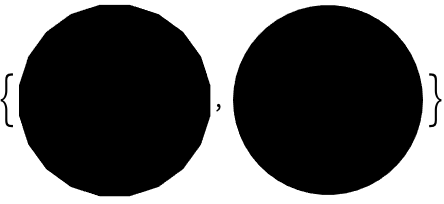Answer & check your solution
+8.3Make a list of polygons with 10, 9, ... , 3 sides. »
Expected output:
 Out[]=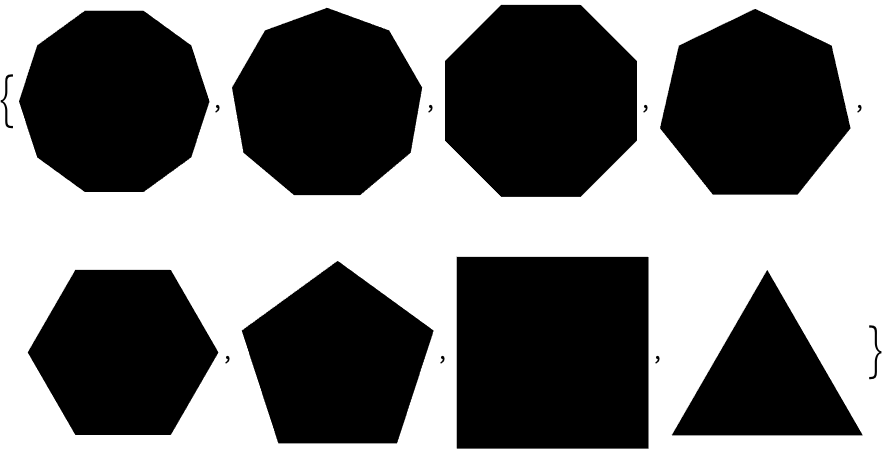Answer & check your solution
How can I make graphics with several objects?
Section 14 will explain. To do so requires understanding coordinates.
Why use Circle[ ], not just Circle?
For consistency. As well see in Section 14, Circle[ ] is actually short for Circle[{0, 0}, 1], which means a circle of radius 1 and center coordinates {0, 0}.
Why isnt the yellow sphere pure yellow?